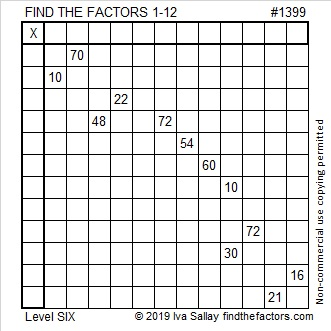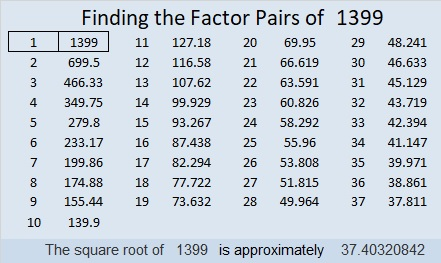# 1399 and Level 6

The eligible common factors of 48 and 72 are 6, 8, and 12. The common factors for 10 and 30 are 5 and 10.  Don’t guess and check the possibilities! Can you figure out the logic needed to start this puzzle?Print the puzzles or type the solution in this excel file: 12 Factors 1389-1403

Here’s a little information about the number 1399:

• 1399 is a prime number.
• Prime factorization: 1399 is prime.
• 1399 has no exponents greater than 1 in its prime factorization, so √1399 cannot be simplified.
• The exponent in the prime factorization is 1. Adding one to that exponent we get (1 + 1) = 2. Therefore 1399 has exactly 2 factors.
• The factors of 1399 are outlined with their factor pair partners in the graphic below.How do we know that 1399 is a prime number? If 1399 were not a prime number, then it would be divisible by at least one prime number less than or equal to √1399. Since 1399 cannot be divided evenly by 2, 3, 5, 7, 11, 13, 17, 19, 23, 29 31, or 37, we know that 1399 is a prime number.

1399 is the difference of two squares:
700² – 699² = 1399

Advertisements